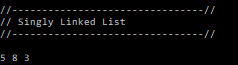A singly linked list is a linear data structure that stores the address or reference of the next node in the list:Singly linked lists are typically used when data is dynamic and changing at runtime. The amount nodes, that can be stored, isn’t a fixed number and can increase in size. This makes it useful to use if there is an unknown number of nodes needed.

#### How to implement a singly linked list

```#include <iostream>

class LinkedList
{
public:
// functions here
private:
typedef struct Node{
int data; // stores the data value
Node* next; // stores address of next node
}* nodePtr;

nodePtr Head = nullptr; // set to nullptr to avoid garbage value
nodePtr Curr = nullptr; // set to nullptr to avoid garbage value
nodePtr Temp = nullptr; // set to nullptr to avoid garbage value
};```

The first thing we want to do is create our Node struct to hold the data of each Node and the next Node along. I am using a typedef here to neaten up the code, alternatively you could write it like this:

```struct Node{
int data; // stores the data value
Node* next; // stores address of next node
};

Node* Head = nullptr;
Node* Curr = nullptr;
Node* Temp = nullptr;```

As you can see, the typedef makes it easier to read and manage. I forgot to mention that the struct is written in private because we don’t need to access these values outside of the class.

Next we need to create some simple functions to call outside of the class

```void AddNode(int addData); // Adds a node to the end of the list
void InsertStart(int addData); // Adds a node to the start of the list
void DeleteNode(int delData);
void PrintList();```

Lets focus on the AddNode function for now, this function will handle putting data at the end of the list

```void LinkedList::AddNode(int addData)
{
nodePtr n = new Node;
n->data = addData; // set the data
n->next = nullptr; // set next to nullptr

if (Head != nullptr) // if head exists
{
Curr = Head; // set curr value to the last node
while (Curr->next != nullptr) //loop through till we get to the end of the list
{
Curr = Curr->next;
}
Curr->next = n; // link happens here
}
else
{
Head = n; // if head doesn't exist, set it
}
}```

If we pass our first value into this function, our Head variable will be set as NULL as we haven’t yet passed a value into it. So we can just set Head = n which sets its int data to the value passed and the next Node to NULL. When we pass in our second value, Head won’t be set to NULL anymore and we can input our second value by setting the Curr variable to the Head variable then traversing through the list until we reach the end (see the while loop) then we link the Node using Curr->next = n

We dont have a way to print out the values we have passed yet so lets work on that next:

```void LinkedList::PrintList()
{
Curr = Head;
while (Curr != nullptr)
{
std::cout << Curr->data << " ";
Curr = Curr->next;
}

}```

The first thing we do is set Curr variable to the first Node in the list (Head). Head will always point to the first Node. Then we can have a while loop to check that the current data isnt NULL. Then in the while loop we just print it to console and set the Curr value to the next Node in the list to print the next value, aslong as it doesn’t return as NULL.

```#include "LinkedList.h"

int main()
{
LinkedList list;
list.AddNode(5); // Add node
list.AddNode(8);
list.AddNode(3);
list.PrintList(); // Print the list
}```

Now we can call a few functions in our main.cpp file. This will output the values 5, 8, 3 (in that order).In the next tutorial, I will be focusing on the InsertStart() and DeleteNode() functions.

Click here to see how to implement a template class with the Linked List (not yet written).